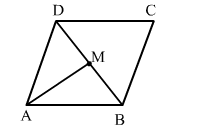# In the given figure, ABCD is a parallelogram, M is the mid-point of BD and BD bisects ∠B as well as ∠D.

Question:

In the given figure, ABCD is a parallelogram, M is the mid-point of BD and BD bisects B as well as ∠D. Then, ∠AMB = ?
(a) 45°
(b) 60°
(c) 90°
(d) 30°Solution:

(c) ​90°

Explanation:
∠B = ∠D

$\Rightarrow \frac{1}{2} \angle B=\frac{1}{2} \angle D$